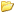Call me back |My basket | Checkout | Add to email listYou are here: Website » Knowledge base

# 1.  Measurement methods

Please note that some of the measurement methods are statistical functions. If you require detailed information on the meaning of the statistical function please consult a simple statistics reference - no explanation of statistics is given here.

## 1.1  "Change In"

This is the change in the value of a variable from the start point to the end point of the measurement.

## 1.2  "Average Of"

This is the average (or mathematical mean) of the variable from the start point to end point of the measurement.

## 1.3  "Maximum Of"

This is the maximum value of the variable from the start point to end point of the measurement.

## 1.4  "Minimum Of"

This is the minimum value of the variable from the start point to end point of the measurement.

## 1.5  "Std Dev Of"

This is the standard deviation of the variable from the start point to end point of the measurement.

## 1.6  "COV Of"

This is the co-efficient of variance (the standard deviation / average, expressed as a percentage) of the variable from the start point to end point of the measurement.

## 1.7  "98% Max Of"

This is the maximum value of the variable between the start and endpoints after the highest 2% of values have been removed. Mathematically this is the 98th percentile of the samples. Note that parameter is calculated using the actual data, and not assuming a normal distribution.

## 1.8  "95% Max Of"

This is the maximum value of the variable between the start and endpoints after the highest 5% of values have been removed. Mathematically this is the 95th percentile of the samples. Note that parameter is calculated using the actual data, and not assuming a normal distribution.

## 1.9  "90% Max Of"

This is the maximum value of the variable between the start and endpoints after the highest 10% of values have been removed. Mathematically this is the 90th percentile of the samples. Note that parameter is calculated using the actual data, and not assuming a normal distribution.

## 1.10  "98% Min Of"

This is the minimum value of the variable between the start and endpoints after the lowest 2% of values have been removed. Mathematically this is the 2nd percentile of the samples. Note that parameter is calculated using the actual data, and not assuming a normal distribution.

## 1.11  "95% Min Of"

This is the minimum value of the variable between the start and endpoints after the lowest 5% of values have been removed. Mathematically this is the 5th percentile of the samples. Note that parameter is calculated using the actual data, and not assuming a normal distribution.

## 1.12  "90% Min Of"

This is the minimum value of the variable between the start and endpoints after the lowest 10% of values have been removed. Mathematically this is the 10th percentile of the samples. Note that parameter is calculated using the actual data, and not assuming a normal distribution.

## 1.13  "Value At Start In"

This is the value of the variable at the start point of the measurement.

## 1.14  "Value At End In"

This is the value of the variable at the end point of the measurement.

## 1.15  "Integral Of"

This is the value of the variable integrated against time from the start point of the measurement.

## 1.16  "Integrated Square Of"

This is the value of the variable squared integrated against time from the start point of the measurement.

## 1.17  "Average Square Of"

This is the average squared value of the variable between the start point and the end point of the measurement interval.

## 1.18  "Range Of"

This is the maximum spread in the data between the start point and the end point of the measurement. It is the equivalent of the "Maximum in the samples - Minimum in the samples".

## 1.19  "Transitions In"

This is the number of changes in the data greater than 0.5. Typically this would be used on "digital" variables such as gear change data etc. It is of very limited use for "analogue" variables such as speed etc.

## 1.20  "Time Positive"

This is the total time that the variable was positive for between the start point and the end point of the measurement.

## 1.21  "Time Zero"

This is the total time that the variable was exactly zero for between the start point and the end point of the measurement. Note that because the variable needs to be exactly zero, it is only of practical use on "digital" variables such as the acceleration/braking flag, not "analogue" variables such as speed.

## 1.22  "Time Negative"

This is the total time that the variable was negative for between the start point and the end point of the measurement.

## 1.23  "99% Max Of"

This is the maximum value of the variable between the start and endpoints after the highest 1% of values have been removed. Mathematically this is the 99th percentile of the samples. Note that parameter is calculated using the actual data, and not assuming a normal distribution.

## 1.24  "99.9 Max Of"

This is the maximum value of the variable between the start and endpoints after the highest 0.1% of values have been removed. Mathematically this is the 99.9th percentile of the samples. Note that parameter is calculated using the actual data, and not assuming a normal distribution.